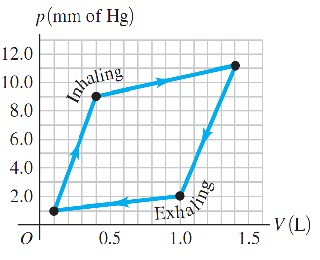# Problem: Work Done by the Lungs. The graph (Figure 1) shows a pV-diagram of the air in a human lung when a person is inhaling and then exhaling a deep breath. Such graphs, obtained in clinical practice, are normally somewhat curved, but we have modeled one as a set of straight lines of the same general shape. (Important: The pressure shown is the gauge pressure, not the absolute pressure.)The process illustrated here is somewhat different from those we have been studying, because the pressure change is due to changes in the amount of gas in the lung, not to temperature changes. (Think of your own breathing. Your lungs do not expand because they've gotten hot.) If the temperature of the air in the lung remains a reasonable 20°C, what is the maximum number of moles in this person's lung during a breath?

###### FREE Expert Solution

The ideal gas equation:

$\overline{){\mathbf{P}}{\mathbf{V}}{\mathbf{=}}{\mathbf{n}}{\mathbf{R}}{\mathbf{T}}{\mathbf{=}}{\mathbf{NkT}}}$n is the number of moles while N is the number of particles.

1mmHg = 133.3N/m2

1L = 10-3m3

At maximum volume,

99% (145 ratings)###### Problem Details

Work Done by the Lungs. The graph (Figure 1) shows a pV-diagram of the air in a human lung when a person is inhaling and then exhaling a deep breath. Such graphs, obtained in clinical practice, are normally somewhat curved, but we have modeled one as a set of straight lines of the same general shape. (Important: The pressure shown is the gauge pressure, not the absolute pressure.)The process illustrated here is somewhat different from those we have been studying, because the pressure change is due to changes in the amount of gas in the lung, not to temperature changes. (Think of your own breathing. Your lungs do not expand because they've gotten hot.) If the temperature of the air in the lung remains a reasonable 20°C, what is the maximum number of moles in this person's lung during a breath?